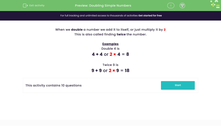# Doubling Simple Numbers

In this worksheet, students double numbers as quickly as possible using the multiplication facts they already know.Key stage:  KS 2

Curriculum topic:   Number: Multiplication and Division

Curriculum subtopic:   Write Multiplication/Division Statements for Known Tables

Popular topics:   Multiplication worksheets

Difficulty level:#### Worksheet Overview

When we double a number we add it to itself, or just multiply it by 2

This is also called finding twice the number.

Examples

Double 4 is

4 + 4 or 2 × 4 = 8

Twice 9 is

9 + 9 or 2 × 9 = 18

### What is EdPlace?

We're your National Curriculum aligned online education content provider helping each child succeed in English, maths and science from year 1 to GCSE. With an EdPlace account you’ll be able to track and measure progress, helping each child achieve their best. We build confidence and attainment by personalising each child’s learning at a level that suits them.

Get started